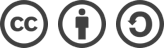## PAT 银行排队问题之单窗口“夹塞”版（队列+模拟）解题报告

2017-03-08 2,447 次浏览

## 参考代码

#include <cstdio>
#include <algorithm>
#include <set>
#include <map>
#include <queue>
using namespace std;

struct info {
char name;
int t;
int p;
} a;

int Hash(char *s) { // 把字符串哈希成数字，防止超时
return (s-'A')*26*26 + (s-'0')*26 + s-'0';
}

int main(int argc, char const *argv[]) {
int n, m, l;
char s;
bool vis = {false}; // 标记是否已办理完毕
scanf("%d %d", &n, &m);
set<int> st; // 朋友圈集合
map<int, int> mp; // 映射人名到朋友圈编号
for(int i=0; i<m; ++i) {
scanf("%d", &l);
while(l--) {
scanf("%s", s);
st[i].insert(Hash(s));
mp[Hash(s)] = i;
}
}
for(int i=0; i<n; ++i) {
scanf("%s %d %d", a[i].name, &a[i].t, &a[i].p);
if(a[i].p > 60) a[i].p = 60;
}
queue<info> q;
q.push(a);
vis = true;
int last = a.t+a.p;
int sum = 0;
while(!q.empty()) {
info f = q.front();
q.pop();
printf("%s\n", f.name);
bool found = false;
for(int i=1; i<n; ++i) { // 寻找一个到达时间不晚于自己办理结束时间的朋友
if(vis[i]) continue;
if(a[i].t > last) break;
if(st[mp[Hash(f.name)]].count(Hash(a[i].name))) {
q.push(a[i]);
vis[i] = true;
found = true;
sum += last-a[i].t;
last += a[i].p;
break;
}
}
if(!found) { // 未找到，则按原顺序找下一个人
for(int i=1; i<n; ++i) {
if(vis[i]) continue;
q.push(a[i]);
vis[i] = true;
sum += max(0, last-a[i].t); // 等待时间可能为 0（窗口空闲一段时间）
if(a[i].t > last) last = a[i].t;
last += a[i].p;
break;
}
}
}
printf("%.1f\n", 1.0*sum/n);

return 0;
}